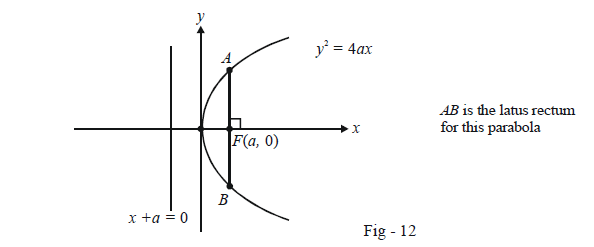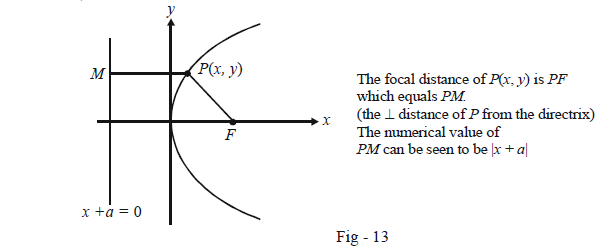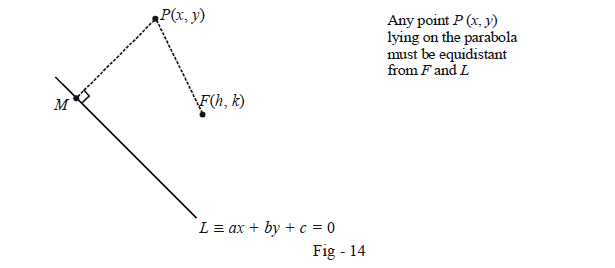# Terminology of Parabolas

Go back to  'Parabola'

We now focus on some important terminology pertaining to parabolas. We will be using the parabola $${y^2} = 4ax$$ as illustration for this purpose; however, this discussion is general.

LATUS-RECTUM: This is a unique chord for a given parabola. It is the chord passing through the focus and perpendicular to the axis of the parabola.The length of the latus rectum can easily be evaluated. Substituting x = a in the equation for the parabola gives two corresponding values for y, i.e., $$y = \pm 2a$$ corresponding to A and B. Thus, the length AB is 4a.

\Rightarrow \quad \boxed {{\begin{align}\begin{array}{l}{\rm{Length\;of \;Latus - Rectum \;of\;}}\\{y^2} = 4ax\end{array}{\;\;\;\;\;\;\; = \;\;\;\;4a}\end{align}}}

From now on, we will abbreviate the latus-rectum as LR. The length of the LR can also be seen to be 4a this way. Since A lies on the parabola, its distance from F, i.e., AF must equal its distance from x +a = 0, which is 2a by inspection. Thus, AB = 2AF = 4a.

FOCAL-CHORD: Any chord passing through the focus of the parabola will be its focal chord. The LR is a particular focal chord.

FOCAL DISTANCE: This, as the name suggests, is the distance of any point on the parabola from its focus, and which will by definition, by equal to its distance from the directrix.Thus,

\Rightarrow\quad \boxed{{\begin{align}\begin{array}{l}{\rm{Focal\;distance\;of\;}}P(x,y)\\ {\rm{on\; }}{y^2} = 4ax\end{array}{\quad =\quad \left| {x + a} \right|}\end{align}}}

We now finally turn our attention to the most general form for the equation of a parabola, i.e., suppose that we are given an arbitrary fixed point F(h, k) and a fixed line $$L \equiv ax + by + c = 0$$ as the focus and directrix of the parabola. What is its equation is such a case?

We use the definition for the parabola, i.e. any point P(x, y) lying on it must be equidistant from F and L.Referring to the figure above, we have

\begin{align}&\qquad \;\; P{M^2} = P{F^2}\\&\Rightarrow \quad\frac{{{{(ax + by + c)}^2}}}{{{a^2} + {b^2}}} = {(x - h)^2} + {(y - k)^2}\qquad\qquad\dots\left( 1 \right)\\& \Rightarrow \quad {a^2}{x^2} + {b^2}{y^2} + {c^2} + 2abxy + 2acx + 2bcy\\&\qquad \qquad \qquad \qquad \quad = ({a^2} + {b^2})\{ {x^2} + {y^2} + {h^2} + {k^2} - 2hx - 2ky\} \\&\Rightarrow \quad {b^2}{x^2} + {a^2}{y^2} - 2abxy - (2h({a^2} + {b^2}) + 2ac)x - (2k({a^2} + {b^2}) + 2bc)y\\&\qquad \qquad \qquad \qquad \quad + ({a^2} + {b^2})({h^2} + {k^2}) - {c^2} = 0 \end{align}

Whatever the coefficients maybe, we see that the equation of a parabola in general has the form

$A{x^2} + 2Hxy + B{y^2} + 2Gx + 2Fy + C = 0 \qquad \qquad \dots\left( 2 \right)$

So that (2) can be expressed in the form (1) (only then can (2) represent a parabola), it can be shown that the coefficients in (2) must satisfy the relations

\Delta = \left| {\begin{align}& A&&H&&G\\ &H&&B&&F\\& G&&F&&C \end{align}} \right| \ne 0 \;\;\;and \;\;{H^2} = AB

This is left to the rigor-bent reader as an exercise

Example – 2

Find the equation of the parabola with focus F(–1, –2) and directrix $$L \equiv x - 2y + 3 = 0.$$

Solution: Assuming P(x, y) to be any point on the parabola, we must have, by virtue of P being equidistant from F and L,

\begin{align}& \qquad \frac{{{{(x - 2y + 3)}^2}}}{5} = {(x + 1)^2} + {(y + 2)^2}\\\\& \Rightarrow \quad {x^2} + 4{y^2} + 9 - 4xy + 6x - 12y = 5{x^2} + 5{y^2} + 10x + 20y + 25\\\\ &\Rightarrow \quad 4{x^2} + {y^2} + 4xy + 4x + 32y + 16 = 0\end{align}

This is the required equation.